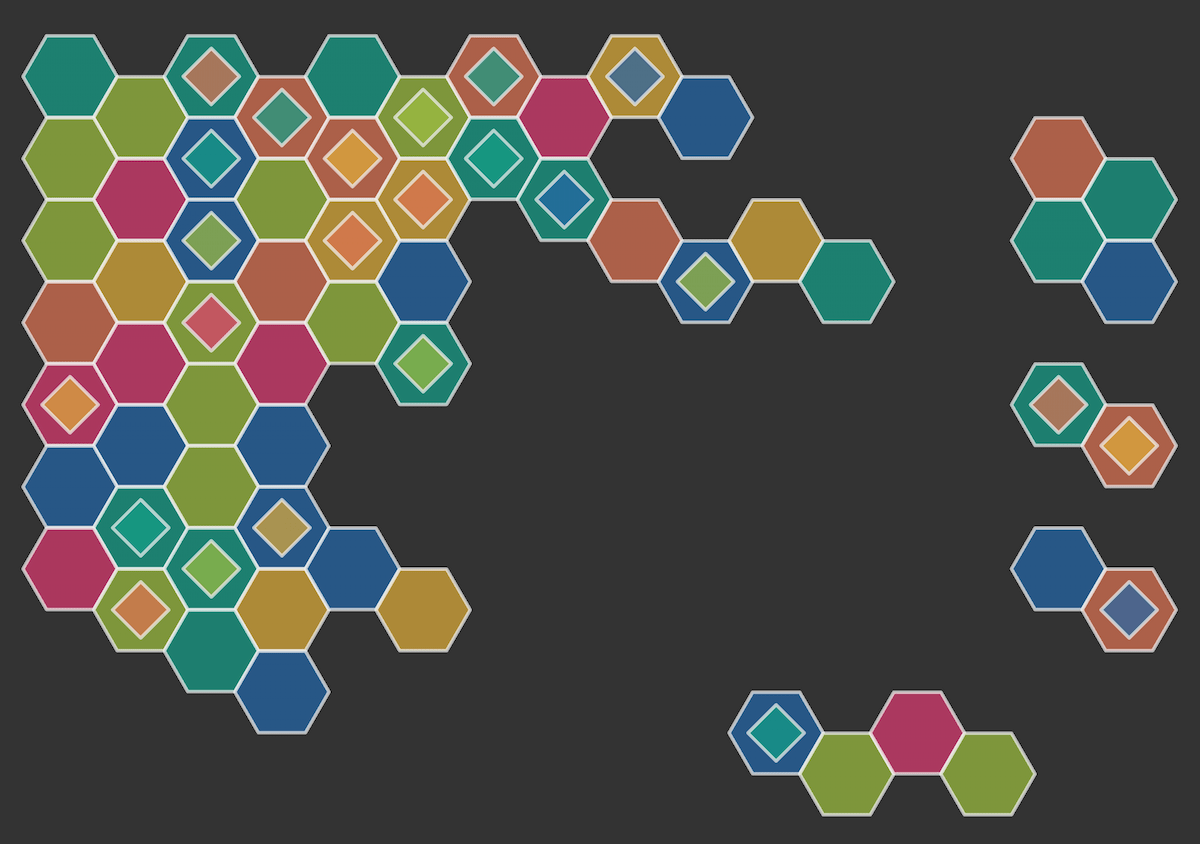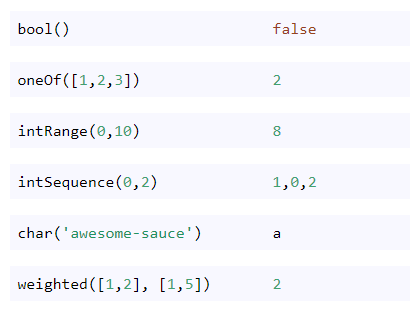第214期 — 2023-06-03 在浏览器中阅读
 周e信Node.jsBetwixt Labs
 Tom Preston-Werner
 BlazityRob Koch
 Olivier Louvignes
 Javascript``````function drawShape(x, y, r, sides) { // move the canvas to the center position ctx.translate(x, y); for (let i = 0; i < sides; i++) { // calculate the rotation const rotation = ((Math.PI * 2) / sides) * i; // for the first point move to if (i === 0) { ctx.moveTo(r * Math.cos(rotation), r * Math.sin(rotation)); } else { // for the rest draw a line ctx.lineTo(r * Math.cos(rotation), r * Math.sin(rotation)); } } // close path and stroke it ctx.closePath(); ctx.stroke(); // reset the translate position ctx.resetTransform(); }``````Ruth John